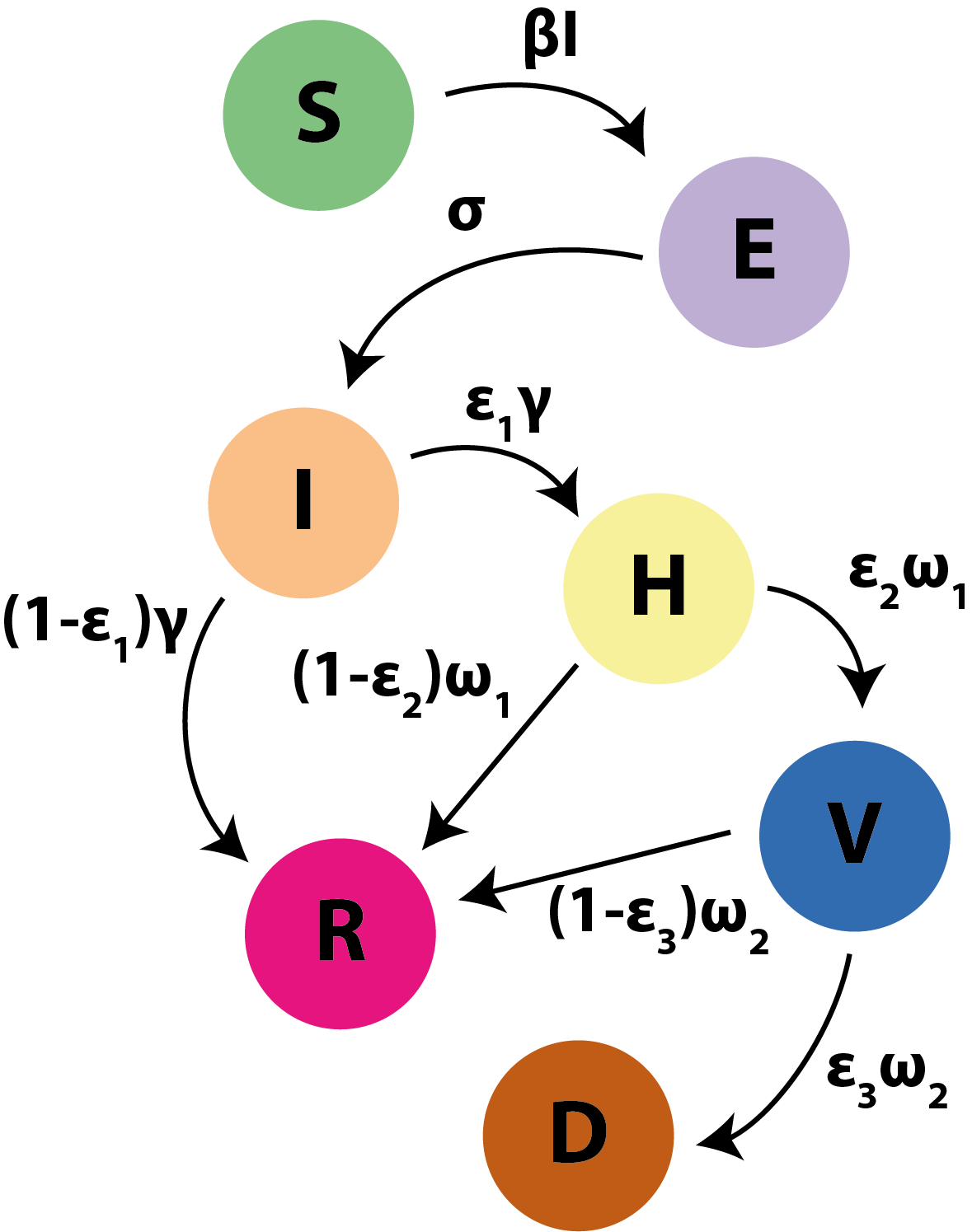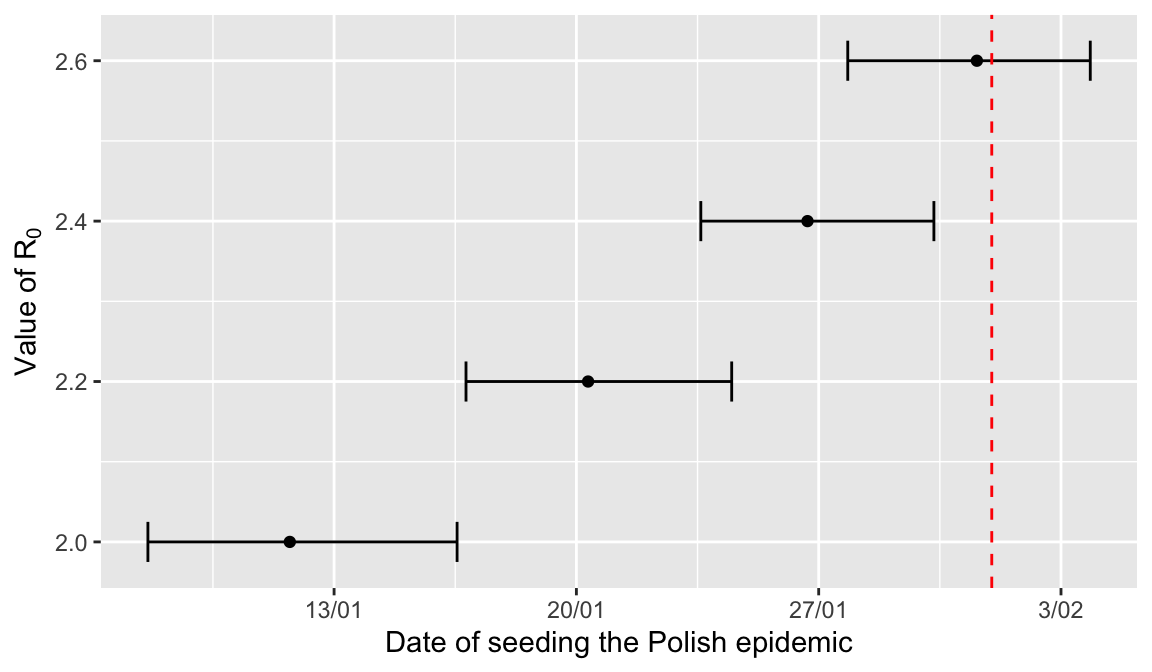# Assessing and predicting epidemiological dynamics of the COVID-19 pandemic in Poland

## Summary

Poland, like other countries, is currently undergoing COVID-19 pandemic, caused by the SARS-CoV-2 virus, however to date few epidemiological predictions of the course of the Polish epidemic have been conducted so far. Here, I use a mathematical model which mimics a SARS-like epidemic and fit it to the reported number of deaths in Poland, as reported by the Ministry of Health, to quantify and predict the epidemiological dynamics in Poland. Specifically, I quantify the true start of the epidemic in Poland, the degree of case under-reporting and attempt to project the epidemic curve in the coming months depending on the efficiency of governmental social distancing measures. Here I summarise the main findings.

• I estimate that the Polish epidemic began sometime around the second half of January. This suggests that the first documented COVID-19 case in Poland on 04/03/2020 is unlikely to be the first infected patient in Poland.

• We currently have a large degree of case under-reporting. I estimate that we are probably missing between 50% and 75% of actual cases, a common phenomenon in many other countries. This number coincides with the previous estimates of the proportion of the population for whom the SARS-CoV-2 infection is asymptomatic or mild.

• The degree of case under-reporting has been declining in recent days, presumably thanks to the increasing number of viral infection tests performed daily. I find that the trend of increasing proportion of cases reported will only continue if social distancing measures will substantially reduce viral transmission.

• The effectiveness of governmental social distancing measures will be crucial for curbing the epidemic in the coming months, helping to prevent tens of thousands of unnecessary deaths.

• Even if thanks to an extreme reduction of viral transmission via social distancing measures the epidemic will fade away in the coming weeks, the majority of the population will be left susceptible, leaving Poland vulnerable to future COVID-19 outbreaks. Given how little we know about the impact of seasonal variation on SARS-CoV-2 transmission, Poland needs a long-term strategy of dealing with the pandemic by preventing future outbreaks until mass vaccination becomes available.

## Introduction

As of today, I have seen few attempts to predict epidemiological dynamics of the COVID-19 pandemic in Poland. The ones I have seen rely on fitting exponential functions to the number of reported cases. This approach has at least two major flaws. The first flaw is that the exponential phase of the epidemic only holds at the beginning when the majority of the population is still susceptible to infection, and hence it cannot be used to assess when the epidemiological curve will slow down and reach a maximum. The second flaw is that, as COVID-19 is thought to be asymptomatic in a large proportion of the population, the number of reported cases will depend not only on the epidemiological dynamics but also on the extent to which people are being tested for the presence of the virus. Hence, the ability to predict the outcome of epidemics in Poland, as in any other country, requires an approach that at the very least can account for both of these factors.

Here I use an approach similar to the one developed by my colleagues at the University of Bern, which is based on the idea of fitting a SEIR epidemiological model to the number of reported deaths in COVID-19 patients, arguing that such cases are unlikely to be missed. I fit this model to the official data provided by the Polish Ministry of Health, kindly collected and publicly provided by Michał Rogalski under this link. These data show that the first reported and confirmed case of the SARS-CoV-2 infection in Poland was on March 4th. The Polish government introduced first restrictions 10 days later on March 14th. Using these tools, I attempted to use mathematical modelling to provide insight into the seeding time of the epidemic, estimate the proportion of undetected cases as of 26/03, and predict different epidemic scenarios depending on the efficacy of the governmental restrictions. I also consider caveats of this approach and comment on limitations of mathematical modelling in epidemiology. Finally, I provide the code written using R Markdown, which anyone is welcome to download and use.

## Methodology

The underlying epidemiological model is based on the classical SEIR approach, with the important difference that it explicitly considers people who are admitted to the hospital, the ICU and those who die from infection. The model can be summarisedFigure 1. Summary of the epidemiological model used. Susceptibles (S) become exposed (E) when they get in touch with an infectious person (I) at a rate $$\beta I$$, and the exposed become infectious at a rate $$\sigma$$. The infecteds recover (R) at a rate $$(1-\epsilon_1)\gamma$$ and become hospitalised (H) at a rate $$\epsilon_1\gamma$$. The hospitalised recover at a rate $$(1-\epsilon_2)\omega_1$$ and get admitted to the ICU (V) at a rate $$\epsilon_2\omega_1$$. The ICU patients recover at a rate $$(1-\epsilon_3)\omega_2$$ and die (D) at a rate $$\epsilon_3\omega_2$$. In this model, $$\beta=R_0\gamma/N$$, where $$R_0$$ is the basic reproduction number and $$N$$ is the population size. The epidemic begins at time $$t_{case}-T_{seed}$$, where $$t_{case}$$ is the date of the first reported case (04/03) and $$T_\text{seed}$$ is the period of time between the first infection and the first actual case. At time $$t_{case}+10$$, namely 14/03, governmental restrictions are introduced at the transmission of the virus is assumed to be $$\kappa R_0$$, where $$\kappa\in[0,1]$$.

I used the existing literature to make assumptions about the parameters used in the model above.

Parameter Value Source
Population size of Poland 38 386 000 Statistics Poland (GUS) Office
Serial interval (latent+infectious period) 7.5 days Li et al.
Duration of hospitalisation for mild and severe cases 8 days Imperial College COVID-19 Response Team: Report 9
Additional duration of hospitalisation for critical cases 8 days Imperial College COVID-19 Response Team: Report 9
Proportion hospitalised cases 5% Adapted from Imperial College COVID-19 Response Team: Report 9
Proportion critical cases 2.5% Adapted from Imperial College COVID-19 Response Team: Report 9
Overall case fatality ratio 1.25% Adapted from Imperial College COVID-19 Response Team: Report 9
Basic reproduction number $$R_0$$ (see here for explanation) 2.0-2.6 Adapted from Imperial College COVID-19 Response Team: Report 9

Table 1. Parameters of the COVID-19 transmission model. The model is fit to the data using a maximum-likelihood approach by comparing the predicted to the reported number of deaths. The daily number of deaths is assumed to be Poisson distributed. Using the parameter values assumed above, we estimate the values of $$T_\text{seed}$$ and $$\kappa$$ that best explain the observed data given the assumed model. We consider four different values of $$R_0=\{2.0, 2.2, 2.4, 2.6\}$$.

## Results

### 0. Importance of the parameter $$\kappa$$ (kappa)

As far as the prediction of the model goes, the value of $$\kappa$$ is the most important parameter to determine the impact of the COVID-19 pandemic in Poland. It represents the impact of the governmental social distancing interventions (so called “lock-downs”) on the viral basic reproduction number $$R_0$$ (number of secondary infections from an infectious person in a fully susceptible population; epidemic grows when $$R_0>1$$ and fades away when $$R_0<1$$). Specifically, $$\kappa=1$$ corresponds to the situation where the interventions have no impact on viral transmission ($$R_0$$ does not change), whereas $$\kappa=0$$ corresponds to the situation where the interventions have complete impact on viral transmission ($$R_0=0$$). Neither of these extremes is likely to be the case, and in reality $$\kappa$$ will lie somewhere in the range between 1 and 0. The purpose of this approach is to estimate $$\kappa$$, however as of 26/03/2020 we do not have enough signal from the data to estimate it: for each of the preassumed values of $$R_0$$, the estimated $$\kappa$$ was close to 1, however this result was not statistically significant (95% confidence intervals were [0,1]).

### 1. When did the Polish epidemic really start?

On the other hand the estimates of $$T_\text{seed}$$ were statistically significant, hence I attempted to estimate the time when the Polish epidemic started (i.e., was “seeded”). The results, shown in Figure 2, suggest that the epidemic we are seeing in Poland began in the second half of January. Please note that this does not mean that the virus physically appeared in Poland then as the true “patient zero” likely contracted the virus abroad and subsequently brought it to Poland. However, this suggests that the virus was most likely circulating in Poland much earlier than when the first case got reported in the media on 04/03.Figure 2. Estimated time of seeding infection in Poland. Bars show the 95% confidence intervals of the estimated time, $$T_\text{seed}$$, translated to actual dates for an assumed value of $$R_0$$. Dashed red bar shows the start of February.

### 2. How many infected people there are in Poland right now?

A major challenge with the COVID-19 pandemic is that likely many people are asymptomatic, at least for a certain period of time, which makes it difficult to detect all cases available. A recent report from London School of Hygiene and Tropical Medicine suggests that in many countries there is a substantial degree of case under-reporting of SARS-CoV-2 infected patients. The number of daily RT-PCR tests carried out in Poland has been increasing each day, but it is unclear whether we have been getting better at countering such under-reporting or not. Using the proposed approach here, I tried to assess the scale and the trend in under-reporting assuming that the model can predict the actual number of cases well. The results are shown in Figure 3. They show that in Poland there exists a considerable degree of case under-reporting, however its magnitude will strongly depend on the efficiency of social distancing measures introduced by the government. I estimate that the reported cases constituted around 6%-8% of all infected people in Poland on 14/03 when such measures were introduced. However, my approach suggests that Poland has been getting better at countering under-reporting as the proportion of detected cases has been increasing. As of 26/03, I find that we have been capturing somewhere between 15% to 57% of all hCoV-infected people, largely depending on the value of $$\kappa$$. This degree of under-reporting is not very surprising given the epidemiology of the disease and the testing capacity in many countries. For example, the LSHTM report mentioned above suggests that as of March 23rd, the proportion cases reported varied between countries from under 20% (eg., Italy, Spain, Turkey, UK, France) to somewhere between 50% and 95% (Germany, South Korea). However, such comparisons should be made with caution as different countries are in different stages of the epidemic and the scale of under-reporting is expected to change (hopefully decrease) over time.

If we assume that in reality the value of $$\kappa$$ lies somewhere between 0.2 and 0.8, then in Poland we should be currently reporting somewhere around 18%-45% of all infected cases. Importantly, I found that the increasing trend in the number of tests performed daily (29700 tests performed on 26/03) may be not enough if transmission has not been substantially curbed. As demonstrated in Figure 3 (middle panel), for values of $$\kappa$$ close to 1, I found that the number of tests per infected person is slowing down or decreasing, depending on the value of $$R_0$$. By contrast, for values of $$\kappa$$ closer to 0 such number has been steadily increasing. Given that we are somewhere in between, it is likely that we have been detecting an increasing proportion of all cases in Poland. For example, if we assume that $$R_0=2.6$$ and $$\kappa=0.5$$ (basic reproductive number has been halved to 1.3 due to lockdowns), then on 04/03 we detected (0.4% of all cases, on 14/03 we detected (7.7% of all cases and on 26/03 we detected (28.8% of all cases. Nevertheless, estimating the efficiency of governmental interventions is necessary to perform a more accurate estimate of the changing trend in case under-reporting over time.